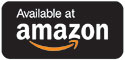Catalog Higher Ed Science and Lab Manuals Ruminant Nutrition System, Vol. II — Tables of Equations and Coding

# Ruminant Nutrition System, Vol. II — Tables of Equations and Coding

## by Luis O. Tedeschi and Danny G. Fox

### Print Version

#### Paperback Price: \$82.86

Paperback ISBN: 978-1-97507-621-4

351 pages

#### Hardcover Price: \$105.71

Hardcover ISBN: 978-1-97507-702-0

Suggested retail price shown above.This is Volume II of a two-volume set. Volume I is available here.

This book accompanies The Ruminant Nutrition System: Volume I — An Applied Model for Predicting Nutri-ent Requirements and Feed Utilization in Ruminants (RNS). It shows the equations and code used to implement the RNS model for use by advanced teaching courses and research by faculty and graduate stu-dents at the master or doctoral levels in life sciences, animal science, wildlife and fisheries sciences, vet-erinary medicine, and biology and zoology, as well as any others who wish to understand the execution of the RNS model.

Since we started writing the first edition of the Ruminant Nutrition System, we planned to include the computer model's equations and the calculation logic. The book, however, quickly became a comprehen-sive document of the published research used to identify essential equations and variables for the under-lying calculation logic of the RNS model. It was a rich, dense source of information about the biology and nutrition of ruminants and the mathematical modeling concepts behind the computer model. As a result, we scattered the RNS model equations throughout the book, within the appropriate chapters containing the pertinent scientific discussions, making it difficult for the reader to reconstruct the computer model. Soon after the publication of the first edition of the book, readers wishing to see the RNS model equations, their linkages, the calculation logic, and how they were implemented into the computer software started requesting more details. To meet this need, we needed to produce a companion book focused on describ-ing the RNS model's equations and code. Before releasing it to the public, however, we had to make sure that the equations accurately reflected the concepts (i.e., the validation step in mathematical modeling) delineated in the RNS's Volume I.

The RNS's Volume II — Tables of Equations and Code arose because of our commitment to document and disseminate the mathematics composing the RNS model clearly and in detail. Each part of Volume II presents the RNS model's equations and the calculation logic in two ways. The first, more traditional approach lists the equations in a tabular form, including an equation number, the independent variable with its description, and a mathematical formulation (in the form of the equation) that follows a logical sequence for calculation and execution. The second approach embeds the equations into a modern, highly aggre-gated method of an actual computer programming language structure, the R script. This second approach presents the sequence and the calculation logic for the equations more systematically and coherently than the first approach for those wishing to understand how the RNS calculations were programmed.